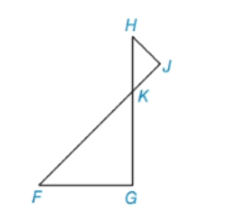Chapter 5.3, Problem 19EElementary Geometry For College St...

7th Edition
Alexander + 2 others
ISBN: 9781337614085

Solutions

Chapter
SectionElementary Geometry For College St...

7th Edition
Alexander + 2 others
ISBN: 9781337614085
Textbook Problem

In Exercises 17 to 24, complete each proof.Given: ∠ H ≅ ∠ F Prove: Δ H J K ∼ Δ F G KExercises 19, 20 PROOF Statements Reasons 1. ? 1. Given 2. ∠ H K J ≅ ∠ F K G 2. ? 3. ? 3. ?

To determine

To complete:

The proof by using given information.

Explanation

Given:

Given: HF

Prove: ΔHJKΔFGK

 PROOF Statements Reasons 1. ? 1. Given 2. ∠HKJ≅∠FKG 2. ? 3. ? 3. ?

Definition:

AA:

If two angles of one triangle are congruent to two angles of another triangle, then the triangles are similar.

Description:

Given that HF.

To Prove: ΔHJKΔFGK

If two angles are vertical angles, then they are congruent. That is, HKJFKG

Still sussing out bartleby?

Check out a sample textbook solution.

See a sample solution

The Solution to Your Study Problems

Bartleby provides explanations to thousands of textbook problems written by our experts, many with advanced degrees!

Get Started

Find more solutions based on key concepts